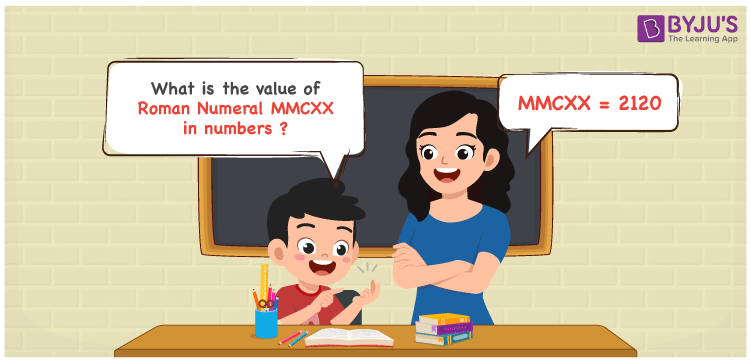Checkout JEE MAINS 2022 Question Paper Analysis : Checkout JEE MAINS 2022 Question Paper Analysis :

# MMCXX Roman Numerals

MMCXX Roman Numerals is 2120. In this article, you will learn the transformation of MMCXX to numbers in a systematic way. Students have to go through the Roman numerals articles given at BYJU’S for a better understanding among the students. Therefore, MMCXX can be indicated as 2120 in numbers.

 Number Roman Numeral 2120 MMCXX## How to Write MMCXX Roman Numerals in Numbers?

Learn the transformation of MMCXX to numbers by going through the steps below.

MMCXX = M + M + C + X + X

MMCXX = 1000 + 1000 + 100 + 10 + 10

MMCXX = 2120

## Video Lesson on Roman Numerals## Frequently Asked Questions on MMCXX Roman Numerals

### Why is the roman numeral of 2120 written as MMCXX?

We know that

MMCXX = M + M + C + X + X

MMCXX = 1000 + 1000 + 100 + 10 + 10

MMCXX = 2120

### Determine the value of 2000 + 120.

We know that

2000 + 120 = 2120

Hence, the value of 2000 + 120 is 2120 which is written as MMCXX.

### What is the remainder we obtain when MMCXX is divided by III?

We know that

MMCXX = 2120

III = 3

So the remainder we obtain when MMCXX is divided by III is II.